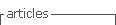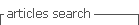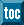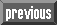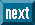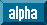## Indicators

•Cited by SciELO
•Access statistics

•Cited by Google
•Similars in SciELO
•Similars in Google

## Print version ISSN 0121-4772

### Cuad. Econ. vol.30 no.54 Bogotá Jan./June 2011

DISTRIBUTION, EXPLOITATION AND PROFIT RATES

To Raquel, fraternally.

Alberto Benítez Sánchez*

* Economics Ph.D. currently occupies the position of professor Universidad Autónoma Metropolitana, Unidad Iztapalapa. Mailing address: San Rafael Atlixco 786, Col. Vicentina, 09840 (México, D. F., México). E-mail:besa@xanum.uam.mx.

Resumen

El artículo estudia la igualdad postulada por Adam Smith entre el precio de cada bien y la suma del ingreso obtenido con su producción. Con este fin, se introduce la tasa de distribución del ingreso para designar la proporción salarios/ganancias correspondiente. Así mismo, después de comparar las teorías de Marx y de Smith se establece que: a) la tasa de distribución del ingreso de cualquier bien es igual a la proporción en la que el precio del mismo (en unidades de salario) excede a su valor y b) la tasa de explotación es una tasa de distribución del ingreso particular, la cual corresponde a la canasta de bienes adquirida con el ingreso de los trabajadores. Finalmente, se encuentra una condición necesaria y suficiente para que la tasa de explotación sea mayor que la de ganancia; y una cota superior no trivial para la cantidad de trabajo incorporado en cada canasta de bienes.

Palabras clave: distribución del ingreso, Marx, Morishima, Smith, tasa de explotación. JEL: C67, D33, E11, E13.

Abstract

This article studies Adam Smith's equality between the price of each good and the income obtained from the good. With this aim, this work introduces the income distribution rate designating the corresponding profit/wage proportion and comparing Marx's and Smith's theories, the article establishes that: a) the income distribution rate of each good is equal to the proportion in which the price of the good (in wage units) exceeds its value and b) the exploitation rate is equal to a particular income distribution rate, the rate corresponding to the commodity bundle acquired with the wage. Moreover, it presents a necessary and sufficient condition for the exploitation rate to be greater than the profit rate and also a non trivial upper bound for the quantities of labor incorporated in any collection of goods.

Key words: exploitation, income distribution, Marx, Morishima, Smith. JEL: C67, D33, E11, E13.

Résumé

L'article analyse l'égalité postulée par Adam Smith entre le prix de chaque bien et la somme du revenu obtenu dans sa production. Dans ce but, on introduit, le taux de distribution des revenus pour désigner la proportion salaires/profits correspondant. De même, après avoir comparé les théories de Marx et de Smith, on établit que : a) le taux de distribution des revenus de n' importe quel bien est égale à la proportion dans laquelle le prix du même bien (en unités de salaire) dépasse sa valeur et b) le taux d'exploitation est donc un taux de distribution de recettes particulier, qui correspond au panier de biens acquis avec le revenu des travailleurs. Finalement, on trouve une condition nécessaire et suffisante pour que le taux d'exploitation soit plus grand que celui des profits ; et une cotte supérieure relevante pour la quantité de travail incorporé dans chaque panier de biens.

Mots clés : distribution des revenus, Marx, Morishima, Smith, taux d'exploitation. JEL : C67, D33, E11, E13.

The economic theories of Adam Smith and Karl Marx share at least two important ideas about the relations between prices and income distribution: (a) in the absence of profit (in Smiths “early and rude state of society”) relative prices are determined by the quantities of labor expended in the production of each good, which I refer to as the value of the good and (b) profit and rent are deductions from labors product, so that their sum changes inversely to wages.To simplify, I will assume in what follows that either there is no rent or that rent is a part of profit. Both coincidences were pointed out by Marx (2000, 77-85) and by Engels (1992, 91-92), although the two authors criticized some of Smiths thesis.

Among said discrepancies, I will only consider here Smith´s equality between the price of each good and the income obtained with its production, criticized by Marx (2000, 97-103). The disaccord rests upon a peculiar aspect of the argument presented by Smith, who observes that the price of each good equals its production cost, which is the sum of the wages, profit, and the price of the means of production consumed to generate the good. As the same is true of the last price, it is possible to equalize the price of each good with the amount obtained adding up the wages and profits of the first two production periods (counting from the most recent one) together with the price of the means of production consumed in the second period. Again, this last price can be substituted by the elements integrating the corresponding production cost and so on. After each substitution the residue, constituted by the price of the means of production, diminishes and tends to disappear1.

Regarding this argument, Marx indicates that any finite number of substitutions will leave a non-zero residue of means of production, so that no matter how far we carry on this process there will always be a part of the capital in the production cost making it impossible for the equality to be accomplished. It may be remarked that the method introduced by Smith shares its basic approach with the “reduction to dated quantities of labor” presented by Sraffa (1960, 34-40).

Nevertheless, in his exposition Sraffa seems to adopt Marx´s position because he considers only a finite number of substitutions, something that allows Roncaglia (2005, 139) to interpret Sraffa´s contribution as proof in favor of Marxs critique2.

Following an original line of reasoning, Dmitriev (1974, 37-45) proves the equality discovered by Smith without any reference to the previous periods of production. In section 3, I study the equality in a linear model of single-product industries,proving its validity by means of a procedure similar to Dmitriev´s but more adequate to the purposes of the article. This permits to obviate the aforementioned controversy and contributes to a better understanding of the relations between prices and income distribution, given the fact that the equality establishes a basic relation between the two concepts that has certain implications.

To highlight this, I define in section 4 the rate of income distribution as the profit/wage proportion corresponding to the total income generated with the production of a commodity bundle. Then, I proceed to establish in section 5 two results that permit the reader to appreciate with greater precision the content of the ideas common to Marx and Smith that I mentioned earlier: 1) the income distribution rate of any commodity bundle is equal to the proportion in which the price (in wage units) exceeds the value of the bundle and 2) the exploitation rate is equal to a particular income distribution rate, the rate corresponding to the commodity bundle acquired by the workers. The first result is a fundamental relation between prices, values, and income distribution, whereas the second one counts among its corollaries. Upon this basis, in the rest of the article I compare with certain detail the exploitation and profit rates.

On this matter, Marx (1991, Ch. 3, 141-142) shows that when wages are paid entirely at the start of production the rate of exploitation (ρ) is always greater than that of profit (r). Although he agrees with this, Morishima (1973, 56-62) presents some objections to the proof offered by Marx and reassumes Marx´s thesis in Morishima and Seton (1961), proving that it is correct in a situation in which workers cannot choose the collection of goods that they consume.

Later he gives a more general scope to this result in Morishima (1973, 65-71), assuming that workers are free to choose the goods that they consume, but under the restriction that they are all homogeneous in tastes. He also indicates that Okisho (1963) reaches an equivalent result to the one established by the two authors just mentioned and for this reason he refers to the thesis as the Morishima-Seton- Okisho Theorem (MSOT)3. On the other hand, Pasinetti (1977, 133) points out that when salaries are paid entirely at the end of production ρ is not necessarily greater than r.

With the intention to investigate the relations between the magnitudes of the two variables in a model compatible with those studied by the authors mentioned in the preceding paragraph, I expose in section 2 a linear model of single-product industries in which a fraction t(0 ≤ t ≤ 1) of salaries is paid in each industry at the beginning of production and the remainder when it finishes.

This permits the inclusion in the analysis of the situations corresponding to the two particular values of t considered in the works already cited as well as all the intermediate situations4.

In section 4, I show that the quantity of labor incorporated in any collection of goods bought with the sum of wages possesses an upper bound that is independent of technology; it is determined solely by r and t. In the next section, I prove that for any non-zero level of the rate of profit compatible with a given production program tr < ρ; it is worth adding that the above-mentioned restrictions are not required so that the last formula is independent of the form chosen by the workers to spend their incomes5. From this result, it follows that the MSOT is valid when t = 1 although, as already mentioned, there are exceptions when t < 1. In section 7, I study the relative magnitudes of the income distribution and profit rates, identifying a necessary and sufficient condition for the MSOT to be valid independently of t. In the next section, I prove that a sufficient condition for the validity of the theorem is that the organic composition of capital in every industrial branch is greater than or equal to 1. In the last section, I present some comments of a general character.

THE MODEL

The model represents a productive system integrated by n industrial branches, each one of them producing a particular type of good labeled by an index i or j, so that i, j = 1, 2, …, n. To simplify, I will refer indistinctly to each one of these labels either as a good or as an index. All the production processes are simultaneous and of equal duration; the quantities of each good are measured with the total quantity produced of the corresponding good and the quantities of salaries with the sum of the salaries paid in the economy. For each pair (i, j) of indexes aij and lj are respectively the quantities of good i and of salaries consumed directly (in the j industry during the period considered) to produce one unit of j. They are non negative quantities such that for every j, aij > 0 for at least one i and lj > 0.

I assume that each quantity of salary pays for an equal quantity of labor so that lj may also be interpreted as the quantity of labor consumed in the j industry. For each j, the price of good j in units of salary is pj and r is the rate of profit of the period. Since 1 − t is the fraction of the wage paid at the end of production, the cost of labor in each branch j is lj t (1 + r) + l j (1 − t) = lj (1 + tr). In these conditions, if the rate of profit is the same in every branch, the prices and costs of production are related by the following system of equations: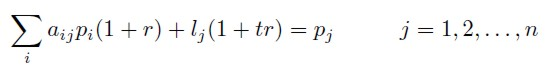A set {j1, j2,…, jd, … , jD} is a D set if it contains D different goods and  is viable if in every D set the sum of the quantities of each good that are consumed directly in the production of D is not greater than 1 and is less than 1 for at least one of the goods. A viable system  possesses the basic properties now indicated.

Theorem 1. There is an interval [0,R[ such that: (a) R is independent of t and 0 < R < +∞, (b) for each r ∈ [0,R[, the solution of  is unique and strictly positive, (c) pj(r) is a monotonous increasing function for every j, (d) at least one price tends to infinity when r tends to R, (e) for each r ∈ [0,R[, the quotient pi(r)/pj(r) is independent of t∀(i, j).

Proof. Propositions similar to (a), (b), (c) and (d) are proved in different publications. A complete proof is presented in Benítez (2009), and some closely related results are also discussed6.

SMITHS EQUALITY

In relation to rent, wages, and profits Smith (1981, Book 1, Ch. VI, 68) states that “In every society the price of every commodity finally resolves itself into some one or other, or all of those three parts; and in every improved society, all the three enter more or less, as components parts, into the price of the far greater part of commodities.” In the present section, I am going to prove this proposition in system .

I will represent with ci a nonnegative quantity of good i and with vector c = (c1 , c2 ,… , cn) a commodity bundle in which ci > 0 for at least one i. To study certain properties of , I will consider some other production programs in which I will represent with xj the quantity produced of good j and with vector x = (x1 , x2 ,… , xn) the commodity bundle produced. Assuming constant returns to scale the production equations of the program producing x may be obtained by multiplying each equation j of  by the corresponding quantity xj , resulting in: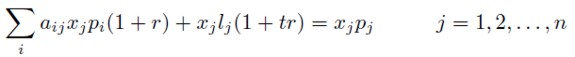I will refer to c as the real income of  if c results after deducting from the quantities produced those consumed as means of production. Also, in this case I

will say that the amounts of income, profits, and wages of  are those generated or obtained with the production of c7. The quantities produced and the real income are related by the following system of equations: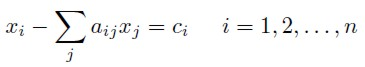Introducing the nxn matrix A = [ aji ], the last system may be written by means of the equation: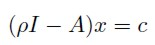where I is the nxn identity matrix and ρ = 1 8. This notation permits to verify the following proposition.

Theorem 2. Every commodity vector c is the real income of a unique system of type .

Proof. Let λA be the Frobenius root of A, the viability of  implies that 0 < λA < 1, as shown in the proof of (a) of Theorem 1. For this reason, B = (ρI − A) satisfies (V I′) of Theorem 4. C. 7 from Takayama (1987, 386). Then, it follows from (I′) of the same theorem together with (I) and (II) of Theorem 4. C. 4 on the same book (Takayama, 1987, 383) that for every c there is an x ≥ 0, verifying , x is unique because B is nonsingular according to ( III ) of the last theorem, ending the proof.

Summing up the n equations of  yields: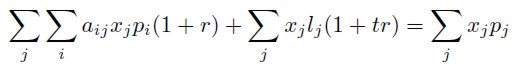From [3[ it follows that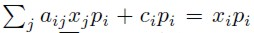for each i and, consequently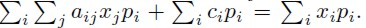. Substituting the right side of  for the left side of the last equation results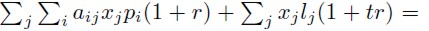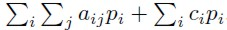. Therefore: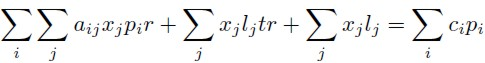The first and second terms of the left side of this equation are the profits obtained respectively with the means of production and the advanced wages while the third one is the amount of wages. The next proposition enounces the meaning of .

Theorem 3. The price of each commodity vector c is equal to the income generated with its production.

In the following sections, I will explore some consequences of this result.

LABOR INCORPORATED

When r = 0 it is possible to write  as the first of the following equations: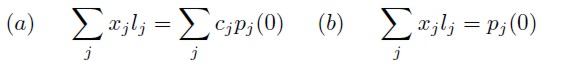The second equation correspond to the system whose real income consist in one unit of j. Given the equality between the quantity of labor and the quantity of salaries paid in each branch, this result proofs for each j that pj(0) is equal to the total amount of labor consumed in the production of the good being considered9. Moreover, I assume that every good being produced is sold in the market so that all the concrete labor is realized as abstract labor. Based on these conditions, I will use the expressions “labor incorporated into j” and “the value of good j” as two indistinct denominations to the corresponding quantity pj(0). On the other hand, for every r ∈]0,R[ the quotient 1/pj(r) is the number of units of good j that can be bought with one unit of salary. As a result, the value acquired indirectly when one unit of salary is spent buying good j is determined by the quotient: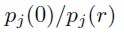This function and (c) of Theorem 1 imply that the labor incorporated acquired is a fraction of the price of the good bought with the wage unit, it depends on r and -as relative prices change when r changes- also on the particular good being bought. I will represent with L(c) the total labor incorporated in a particular c. Therefore, if the cost of c is equal to one wage unit we have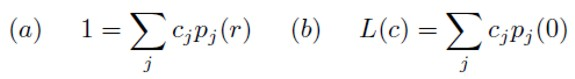There is an upper bound to L(c) indicated in the next proposition.

Theorem 4. If the price of a commodity vector c is one unit of salary then, for any given r ∈]0,R[: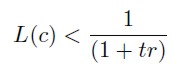Proof. Given any r ∈]0,R[ the greatest quantity of labor incorporated in a commodity vector c whose price is one unit of salary is equal or less than max {pj(0) / pj (r)j = 1, 2,… , n}. For this reason, it is enough to prove that for every j the following inequality holds: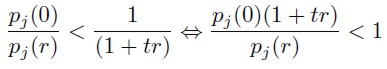Substituting in the denominator of the left side of this inequality the price of good j for its equivalent in  we have: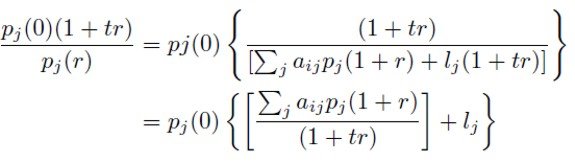On the right side of the last equation all terms are constants except Σ j ai jpj(1 + r)/(1+tr), which is a monotonous increasing function of r because each price is that type of function according to (c) of Theorem 1, as well as (1 + r)/(1 + tr)(for t < 1, otherwise it is constant) and, besides, aij > 0 for at least one i. Consequently, the denominator in that side is an increasing function of r for each r ∈]0,R[. This conclusion and the fact that this quotient is equal to 1 when r = 0 permits to verify , ending the proof of the Theorem.

The following proposition gives a more general expression to the basic idea in Theorem 4.

Corollary to Theorem 4. For any given r ∈]0,R[ and for any commodity vector c: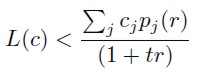Proof. Given c, let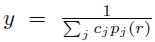, remark that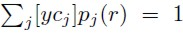. Then, according to Theorem 5 we have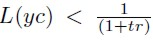, this result and the fact that yL(c) = L(yc) imply that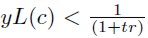so that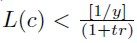. Substituting y for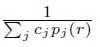in this inequality and simplifying completes the proof.

A REFORMULATION OF THE MSOT

Marx distinguishes the necessary labor time, which is the labor time destined to produce the goods consumed by the workers, from the surplus labor time destined to produce the rest of the goods. Then he defines the rate of exploitation as the quotient of the second quantity divided by the first one. Consequently, if the workers acquire with their salary a particular commodity vector c the necessary labor time is L(c), 1−L(c) is the surplus labor time and the corresponding rate of exploitation ρ is determined by the formula10: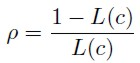In this manner, ρ measures the quantity of time destined to produce profits per unit of time expended producing wages. It also indicates the distribution of the labor time among the goods produced according to the social class that consumes each good. A relation between the magnitudes of ρ and r is established in the following proposition.

Theorem 5. In system , tr < ρ for every r ∈]0,R[..

Proof. Equation  may be written in the form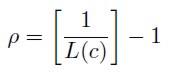From  it follows that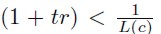so that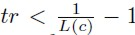. This formula together with  implies that tr <ρ, finishing the proof.

According to this theorem r <ρ when t = 1, as stated in the MSOT11. In section 7 I will establish a necessary and sufficient condition for the theorem to be valid for any t.

EXPLOITATION AND INCOME DISTRIBUTION RATES

For each commodity vector c, I define the income distribution rate S(c) associated to c as the proportion between the profits and the wages obtained with the production of c12. Accordingly, it follows from  that: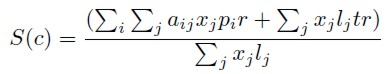I also define the variation rate of the price of c in wage units V (c) as the proportion in which the price of c exceeds its value. Therefore: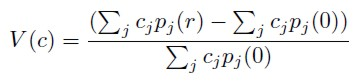The two concepts are related in the following proposition.

Theorem 6. S(c) = V (c) for each commodity vector c andr ∈ [0,R[.

Proof. Equations  and [7.a] imply that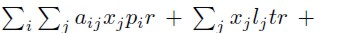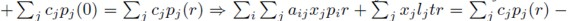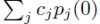. This equation shows that the numerators of  and  are equal, which is also the case with the denominators, according to [7.a].

The theorem establishes an important relation showing that prices increase over values in a rate equal to the corresponding profits/wages proportion. In the rest of the article I am going to consider two implications of this result that are now to be presented.

The following proposition may be verified substituting V(c) for S(c) in  and simplifying its left side.

Corollary 1 to Theorem 6. For every c andr ∈ [ 0,R [: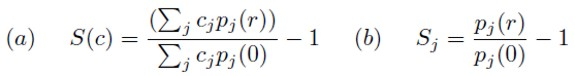the second formula corresponding to the special case when c contains only one unit of a particular good j.

These equations permit the reader to determine any one of the three concepts involved in the corresponding formula as a function of the two others. The price of any commodity vector c measured in wage units was defined by Smith as the labor commanded by c so that (16.a) determines the rate at which the commanded surpasses the incorporated labor in c. However, it is convenient to indicate that the last interpretation is subject to the existence of a single proportion between quantities of labor and quantities of wages already assumed in this article, while the definition of the distribution rate does not require this hypothesis. In the general case the last one is equal to the rate at which the labor commanded by c surpasses the total income obtained by labor in the production of c. On the other hand, the income distribution and exploitation rates are related in the following proposition.

Corollary 2 to Theorem 6. The exploitation rate is equal to a particular income distribution rate, the rate corresponding to the commodity vector acquired by the workers.

Proof. If a vector c is chosen by the workers it determines ρ in . On the other hand, because its cost is one wage unit c verifies [9.a], dividing each side of this equation by the corresponding side of [9.b] yields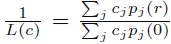. Then,substituting in the right side of [16.a] the quotient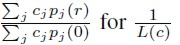results the right side of . Therefore, for this particular c we have S(c) = ρ, ending the proof.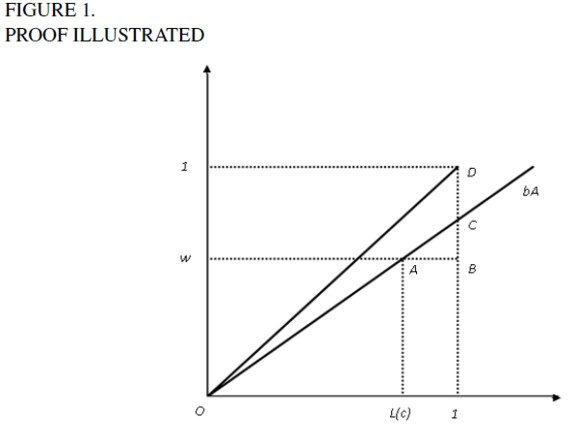Source: own estimations.

This result is illustrated in Figure 1. There, the vertical axis indicates prices measured with the real income of  and the horizontal axis quantities of labor incorporated in the commodity bundles now to be described. The second coordinate (y) of each point on segment OD is the price of a bundle integrated by y units of each good. Let c be the commodity vector acquired by the workers when their wage is equivalent to the fraction w of the real income, the corresponding point in the figure is A = (L(c),w). Therefore, the second coordinate of each point on the straight line bA is the price of the commodity vector bc (remark that b = 1 in A). The income distribution and exploitation rates of  are respectively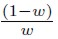and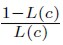, as shown in the figure they are not necessarily equal. On the other hand,the second coordinate of C is the price of the commodity vector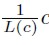, incorporating one unit of labor. The income distribution rate of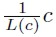and consequently, of c is CB/B1. Indeed, for each r ∈ [0,R[ prices are the same in  an in the system  producing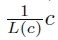as real income. Then, because each system employs one unit of labor, the wage is equivalent to B1 in both systems. The proportion CB/B1 is equal to the exploitation rate given the fact that triangles ABC and OL(c)A are similar.

COMPARING EXPLOITATION AND PROFIT RATES

The Corollary 2 to Theorem 6 implies that ρ is determined only after the sum of wages has been spent. Also, from this conclusion it follows that the difference ρ − r is as a particular case of the functions S(c) − r. These functions can be expressed by means of  in the following forms: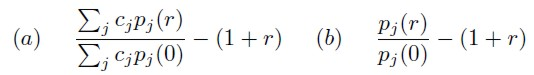The two groups of formulas are related in the following proposition.

Lemma 1. Given an r ∈] 0,R [ a necessary and sufficient condition for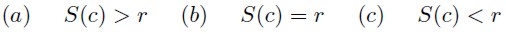For every commodity vector c costing one wage unit is that for every j respectively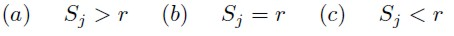Proof. If [19.a] holds it follows from [17.b] that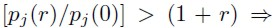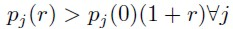and for this reasonConsequently,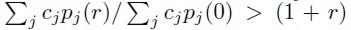and according to [16.a] we have [18.a] verified so that [19.a] ⇒[18.a]. For each j, let c [j] be the commodity vector containing the quantity of good j worth 1 wage unit and no other good. Then, S(c [j]) = Sj for every j so that [18.a] ⇒ [19.a]. The proofs for [18.b] ⇔ [19.b] and [18.c]⇔ [19.c] are closely similar.

It follows from Lemma 1 and Corollary 2 to Theorem 6 that some meaningful consequences about the relation between ρ; and r may be obtained from the study of those cases in which the whole salary is spent buying a single good. This will be done in the rest of the section.

Let KSc and wc represent respectively the price of the means of production and the amount of wages in the system  whose real income is c, measured with the sum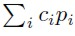. Then: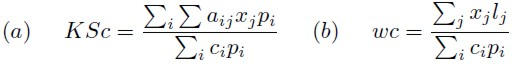Dividing both sides of  by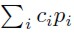and making the substitutions according to  results: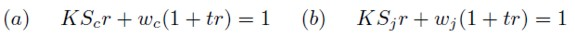The second formula corresponds to the special case when the real income contains only one unit of a particular good j. As in this case total profits and wages, measured with the real income, are respectively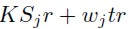and wj the income distribution rate is equal to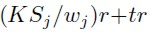so that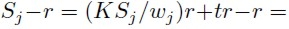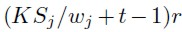. Consequently, the sign of the difference Sjr is the same as that of the function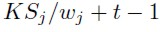. This result and Lema 1 imply the following proposition.

Theorem 7. Given an r ∈]0,R[ a necessary and sufficient condition for [18.a], [18.b], and [18.c] to be verified for every commodity vector c costing one wage unit is respectively that, for every j: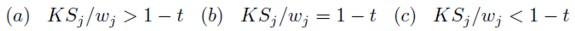In order to explore the economic meaning of this result it is convenient to multiply both sides of each proposition in  by wj and to add twj to each side obtaining the equivalent propositions: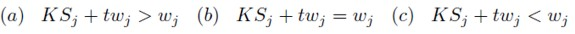Consequently, we can establish the following conclusion:

Corollary to Theorem 7. A necessary and sufficient condition for the exploitation rate to be greater, equal, or less than the rate of profit regardless of the spending of the wage is that the volume of total wages paid in the production of each good is respectively smaller, equal, and greater than the amount of capital invested in the same process.

It is worth noticing that for any commodity vector c the amount of profits of the corresponding system of type  is equal to 1−wc so that the income distribution rate may be calculated by the following formula: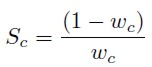On the other hand, it follows from [21.a] that: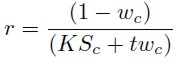The last two equations show that the income distribution rate and the profit rate are equal to total profits divided respectively by the amounts of wages and of the capital advanced. For any r ∈]0,R[ we can divide each side of  by the corresponding side of  obtaining: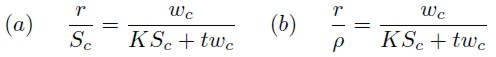The second equation corresponds to the particular c bought by the workers. These formulas are similar to the one presented by Marx (1991, Ch. 3, 142) and show that the proportion between the profit and the income distribution rates (the exploitation rate in the second case) is equal to the proportion between the wage and the amount of capital advanced. For this reason, ρ > r for every r ∈]0,R[ if t = 1 but if t < 1 the opposite relation is possible provided that KSc is small enough. It must be stressed that the second formula is valid only for the particular c bought with the wage while the first one is valid for any c.

SCOPE OF THE MSOT

I present in this section some implications of the previous results. Among them, I show that a sufficient condition for the MSOT to be valid is that the organic composition of capital be greater or equal to 1 in every industrial branch.

Proposition 1. A necessary and sufficient condition for ρ > r in system  for every r ∈; ]0,R[, regardless of the goods bought by the workers, in the following cases: (1) for any t ∈ [tx, 1] where tx is the given value of t in  and 2) for every t ∈ [0, 1], are respectively that KSjwj(1 −tx) and KSjwj when r = 0 for every j.

Proof. As ρ is a particular distribution rate [22.a] is a necessary and sufficient condition for the corollary to be verified in the first case for a given r ∈]0,R[ and for tx. It follows from (c) of Theorem 1 that the left side of [22.a] is a monotonous increasing function of r so that if KSjwj(1 − tx) when r = 0 for every j then [22.a] is verified for every r ∈]0,R[. According to (e) of the same theorem, KSj is independent of t while (1 − t) is a monotonous decreasing function of t so that in this case it is also verified for every t ∈ [tx, 1], proving the proposition in case 1). As the previous result is valid for tx = 0 it implies that the corollary is valid in the second case, ending the proof.

It is important to remark that for each j, KSj and wj result from the sum of several equations. For this reason, in the corresponding system  there may be some equations where the price of the means of production is less than the amount of wages, with the difference being compensated in [21.b] by other equations with an inverse relation between the two quantities. It is then useful to consider the following result:

Proposition 2. A sufficient condition in order that ρ > r for every r ∈]0,R[ and for every t ∈ [0, 1] in system  is that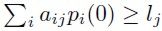for each j.

Proof. The proportion between the price of the means of production and the amount of wages in a branch j is not altered when the jth equation of  is multiplied by an xj,/ > 0, for this reason it is preserved in the corresponding equation of every system of type . As xj > 0 for at least one j, if the condition of the proposition is satisfied we have KSjwj for every j when r = 0, verifying the condition required in the case (2) of Proposition 1.

Marx (1990, Ch. 25, 762) defines the organic composition of capital as the proportion between the value of the means of production invested and the value of the capital advanced to pay wages in a production process. In his analysis he considered t = 1 so that this proportion is equal to KSj/wj when r = 0 because, as proved in section 4, the value of a good is equal to its price when r = 0. Therefore, this proposition shows that a sufficient condition for ρ; > r independently of t, of the particular goods bought by the workers and of the level of r ∈]0,R[ is that the organic composition of capital in each industrial branch is greater than or equal to 113.

Proposition 3. Given a particular technology there is a tx ∈ [0, 1[ such that ρ > r for every r ∈]0,R[ and for every t ∈ [ tx, 1 ].

Proof. Let KSd /wd = min {KSj/wj | j = 1, 2, … , n} when r = 0. If KSd / wd ≥ 1 then tx,/ = 0 according to case (2) of Proposition 1. Otherwise tx = 1− KSd /wd according to case (1) of the same proposition.

Proposition 4. The equation ρρ = r is valid for at least one r ∈]0,R[ if the three following conditions are satisfied: (a) the commodity vector c bought by the workers is a continuous function of r, (b) [19.c] is verified for an ra ∈]0,R[, and (c) [19.a] is verified for an rb ∈]0,R[.

Proof. In these conditions, [18.a] and [18.c] are verified respectively for rb and ra while (a) implies that ρ is a continuous function of r. Also, ρ = S(c) so that ρ < r when r = ra and ρ > r when r = rb. Therefore, ρ = r for at least one r ∈]ra, rb[.

A sufficient condition for (a) is the continuity of the individual demand functions with respect to prices, for (b) an organic composition of capital of less than one unit in every industrial branch (this can be proved with an argument closely similar to the proof of Proposition 2), and for (c) that every good participates either directly or indirectly in the production of each good. Indeed, it follows from (d) of Theorem 1 that in this last case every price (and consequently ρ) tends to infinity when r tends to R.

These arguments suggest that the equality between the two rates may not be a rare occurrence in model . If that is the case r may be substituted by ρ in each equation without changing the price system. This means that the exchange relation between the means of production and the real income is determined by the quantities of labor incorporated in each commodity bundle. But this is not necessarily the case of the other relative prices.

Finally, I will illustrate some of the results by means of the following exercise.

Problem 1. Given wx ∈]0, 1[ and tx ∈ [0, 1[ to find a production system such that r > ρ, r = ρ and r < ρ respectively when w > wx, wx = wx and w < wx.

Solution. I will consider an economy producing one unit of a certain good consuming a11 units of the same good and one unit of labor. As there is only one good the exploitation rate is equal to the income distribution rate of the only good. According to Theorem 7, this equality implies condition (22.b), which can be written in this case as a11/(1 − a11)wx = (1 − tx). Moreover, as the left side of this equation is a monotonous decreasing function of w it results that (22.a) and (22.c) are verified respectively if w < wx and w > wx. In this manner, the problem is reduced to find the value of a11 satisfying the preceding equation. Solving it yields: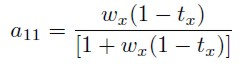For instance, if wx = 8/10 and tx = 1/10, substituting tx and wx for the given quantities results.

a11 = (8/10)(9/10)/[1 + (8/10)(9/10)] = 72/172. Therefore, in the system: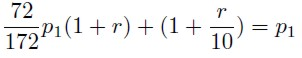ρ is greater than, equal to, and less than r when w is respectively less than, equal to, and greater than 8/10 and less than 1. On the other hand, KS = (72/172)p1/[p1− (72/172)p1] = 72/100, substituting KS and tx with their corresponding values in (25) yields: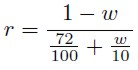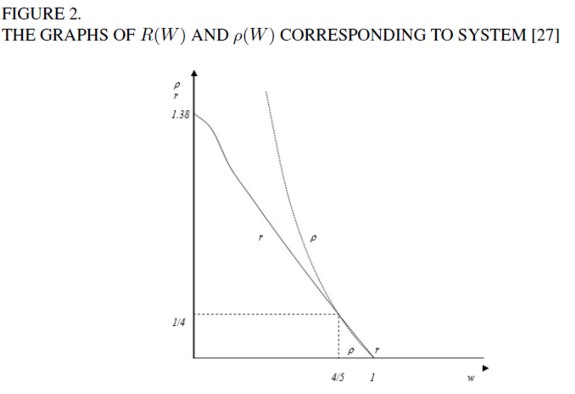Source: own estimations.

The profit rate that is equal to the exploitation rate in  can be calculated substituting w with 8/10 in , obtaining r = ρ = 1/4. Also, when w = 0 equation  permits the reader to establish that R = 100/72, which is approximately equal to 1.39; Figure 2 shows the graphs of  and . Theorem 5 may be also verified: multiplying both sides of  by 1/10 results (1/10) r = (1 − w) / [72/10 + w], this equation and  imply that (1/10) r < ρ for every r ∈]0,R[.

As shown by several publications, it is possible to develop more than one definition of value based upon different passages from the works of Marx and Smith14. Facing this challenge, I adopted definitions that are justified by the original texts, with no pretence to consider them the only valid ones. In my opinion, they provided me with sufficient grounds to advance the analytical task independently of researches trying to select the most appropriate definitions from a hermeneutical perspective. Nevertheless, these researches may eventually offer alternative ways to understand the meaning of the comparative work.

The conclusions (1) and (2) announced in the introduction help to appreciate the proximity of the work of the two authors, which in my view is a necessary complement to the research that tries to distinguish the original contributions of each one15. On this regard, it may be remembered that Marx spent much energy on identifying the original contributions of many authors, including his own ones, but also showed interest in recognizing the collective and cumulative aspects of the scientific work16. According to Schumpeter (1994, 182), Smiths interest on these topics was more restricted17.

NOTAS AL PIE

1 Marx quotes and comments on several passages from Smith (1981), among them the following from page 64 Book 1, Chapter VI: “In that early and rude state of society which precedes both the accumulation of stock and the appropriation of land, the proportion between the quantities of labour necessary for acquiring different objects seems to be the only circumstance which can afford any rule for exchanging them for one another.” And from the next page “The value which the worker adds to the materials, therefore, resolves itself into two parts, of which the one pays their wages, the other the profits of their employer upon the whole stock of materials and wages which he advanced.” He also quotes from pages 83, Book 1, Chapter VIII: “As soon as land becomes private property, the landlord demands a share of almost all the produce which the labour can either raise, or collect from it. His rent makes the first deduction from the produce of the labour which is employed upon land. It seldom happens that the person who tills the ground has wherewithal to maintain himself till he reaps the harvest. His maintenance is generally advanced to him from the stock of a master, the farmer who employs him, and who would have no interest to employ him, unless he was to share in the produce of his labour, or unless his stock was replaced to him with a profit. This profit makes a second deduction from the produce of the labour which is employed upon land.” Regarding the first passage, Marx´s commentary is: “That is to say, the labour-time necessary to produce different commodities determines the proportion in which they exchange for one another, or their exchange-value”. About the third one, he writes that: “Here therefore Adam Smith in plain terms describes rent and profit on capital as mere deductions from the workmans product or the value of his product, which is equal to the quantity of labor added by him to the material. This deduction, however, as Adam Smith has himself previously explained, can only consist of that part of the labour which the workman add to the materials, over and above the quantity of labour which only pays his wages, or which only provides an equivalent for his wages; that is, the surplus labour, the unpaid part of his labour.”

2 “The adding-up-of-components theory, however, does not constitute an adequate solution to the problem of exchange values, science the residual of means of production cannot in general be reduced to zero.” Also, in note 45, in the same page: “Strictly speaking, complete reduction is only possible when no commodity is directly or indirectly required for the production of itself: cf. Sraffa 1960, pp. 34 ff.” In contraposition, Kurz (1983, 606) affirms that: "The proposition that the price of every commodity resolves itself into wages and profits was proved by Sraffa for an economy with single-product industries by means of the reduction to dated quantities of labor method.” This interpretation is shared by Christensen (1979).

3 In pages 63-65 of the book already cited, Morishima refers to the thesis commented here as the MSOT, but in page 6 he uses the same reference for a different proposition, one that he calls the Fundamental Marxian Theorem on page 53.

4 Kliman (2007) criticizes the interpretation of Marxs concepts by means of linear equations systems, the so called simultaneist approach. Nevertheless, I think that a general theory of capitalism, like Marxs, may be studied in the simplest situations (for instance those in which prices are constant along the production period), which are covered by the aforementioned models.

5 As pointed out by Roemer (1993, 33), in advanced capitalism “… workers in fact do choose to consume different bundles and are not limited to subsistence in any meaningful sense”.

6 The original text in Spanish and its English translation may be downloaded respectively at http://redalyc.uaemex.mx/scr/inicio/investigacion economica and http://revistasuam/denarius/index.php.

7 Pasinetti (1977, 134) points out that when wages are paid at the beginning of production they are not included in the classical notion of net product. Following this remark, to avoid confusion, I introduced the “real income”, which is equal to the net product calculated in physical terms when t = 0. For other values of t the two collections of goods would be different.

8 A is the transpose of the coefficient matrix [aij ] integrated by the technical coefficients of .

9 Other proofs of this statement may be found in Sraffa (1960, 34-35), Pasinetti (1975, 89-92) and in Morishima (1973, 10-27).

10 The following three quotations come from Chapter 9, of Marx (1990). “We have seen that the worker, during one part of the labor process, produces only the value of his labour-power, i. e. the value of his means of subsistence [… ] If the value of his daily means of subsistence represent an average of 6 hours objectified labour, the worker must work 6 hours to produce that value [… ]. I call the portion of the working day during which this reproduction takes place necessary labour time and the labour expended during that time necessary labour[…]”(pp. 324-325). “During the second period of the labour process, that in which his labour is no longer necessary labour […] He creates surplus-value which, for the capitalist, has all the charms of something created out of nothing. This part of the working day I call surplus labour time, and to the labour expended during that time I give the name of surplus labour[…]”(p. 325). “In other words, the rate of surplus value, s/v = (surpluslabour) / (necessarylabour) (p. 326). Morishima” (1973, 46-51) exposes three different (but equivalent) definitions of ρ , among them the one adopted here.

11 Mirowski (1988, 171-188) criticizes Morishima´s treatment of fixed capital and other aspects of his work, but not his model of circulating capital. About this he writes on page 187: “Morishimas ‘Fundamental Marxian Theorem (which asserts that exploitation of laborers by capitalist is necessary an sufficient for the existence of a set of prices and wages yielding positive profits) and his solution to the dynamic transformation problem, while admittedly the most original and substantial achievement of the entire book, do not qualify has positive achievements, since Morishima has maintained that the labor theory of value should be abandoned.” Then, according to Mirowski, the achievement (and consequently, the method followed) is correct, although it may be underestimated if the labor theory of value should be abandoned.

12 Shaik (1984) calls this proportion “integrated profit-wage rate”.

13 As is frequently the case with Marxian concepts the organic composition of capital is also subject to different interpretations, as can be appreciated in Steedman (1978, 132-136).

14 For instance, Schumpeter (1994, 188) identifies three different labor-value theories in Smith (1991). Different views about Marx´s concept of abstract labor are confronted in Steedman et al. (1981), Mohum (1994), and in Freeman et al. (2004).

15 Meek (1977) explores both, coincidences and oppositions between the two theorists. Among his conclusions, he states: “Then again, Smith did in certain context come quite close to reducing surplus value to labour, or at any rate to the difference between two quantities of labour the quantity required to bring the annual product to market, and the greater quantity which the annual produce will normally purchase or command. This is not the same as Marxs concept of surplus value by any means, but is nevertheless surely recognizable as a species of the same genus.” (p. 8). The Corollary 2 to Theorem 6 identifies income distribution rates as the genus just mentioned.

16 The second attitude is shown, for instance, in the Postface to the Second Edition, Marx (1990, 99): “ As early as 1871, N. Sieber, Professor of Political Economy in the University of Kiev, in his work David Ricardos Theory of Value and of Capital, referred to my theory of value, money and capital as in his fundamentals a necessary sequel of the teachings of Smith and Ricardo. What astonishes a Western European when he reads this solid piece of work is the authors consistent and firm grasp of the purely theoretical position”.

17 “He was conscientious, painstaking to a degree, methodical, well poised, honorable. He acknowledged obligations where honor required it, but not generously. He never uncovered the footprint of predecessors with Darwinian frankness. In criticism he was narrow and ungenerous. He had the courage and energy that exactly fit the scholar´s task to go well with a good deal of circumspection” (Schumpeter, 1994, 182).

REFERENCES

 Benítez, A. (2009). El pago del salario. Investigación Económica, 68, 270, 69-96.        [ Links ]

 Christensen P. (1979). Sraffian Themes in Adam Smith Theory. Journal of Post Keynesian Economics, 2, 94-109.        [ Links ]

 Dmitriev, V.K. (1974). Economic Essays on Value, Competition and Utility. Cambridge: Cambridge University Press.        [ Links ]

 Engels, F. (1992). Preface to the Second Edition. In Marx, K. Capital, II. New York: Penguin Books.        [ Links ]

 Freeman, A., Kliman, A. and Wells, J. (2004). The new value controversy and the foundations of economics. London: Edward Elgar Publishing Limited.        [ Links ]

 Kliman, A. (2007). Reclaiming Marxs Capital. Plymouth: Lexington Books.        [ Links ]

 Kurz, H.D. (1983). Smithian Themes in Piero Sraffas Theory. In Cunningham, J., Piero Sraffa: critical assessment. London: Croom Helm.        [ Links ]

 Marx, K. (1990). Capital, Volume I. New York: Penguin Books.        [ Links ]

 Marx, K. (1991). Capital, Volume III. New York: Penguin Books.        [ Links ]

 Marx, K. (1992). Capital, Volume II. New York: Penguin Books.        [ Links ]

 Marx, K. (2000). Theories of Surplus Value. New York: Prometheus Books.        [ Links ]

 Meek, R.L. (1977). Smith, Marx, & After. London: Chapman & Hall.        [ Links ]

 Mirowski, P. (1988). Against Mechanicism. Boston: Rowman & Littlefield Publishers, Inc.        [ Links ]

 Mohum, S. (1994). Debates in Value Theory. New York: St. Martin Press Inc.        [ Links ]

 Morishima, M. (1973). Marxs Economics. Cambridge: Cambridge University Press.        [ Links ]

 Morishima, M. and Seton, F. (1961). Aggregation in Leontiefs Matrices and the Labor Theory of Value. Econometrica, 29, 203-220.        [ Links ]

 Okisho, N. (1963). A Mathematical Note onMarxian Theorems. Weltwirtshaftliches Archiv, bd 91, 287-299.        [ Links ]

 Pasinetti, L.L. (1977). Lectures in the theory of production. New York. Columbia University Press.        [ Links ]

 Roemer J.E. (1993). Analytical Marxism. Cambridge: Cambridge University Press and Editions de la Maison de LHomme.        [ Links ]

 Roncaglia, A. (2005). The Wealth of Ideas. Cambridge: Cambridge University Press.        [ Links ]

 Schumpeter, P. (1994). History of Economic Analysis. New York: Oxford University Press.        [ Links ]

 Shaik, A.M. (1984). The Transformation from Marx to Sraffa. In Mandel, E., Freeman. M. (eds.), Ricardo, Marx, Sraffa: The Langston Memorial Volume (pp. 43-84). London: Verso.        [ Links ]

 Smith, A. (1981). An inquiry into the nature and causes of the wealth of nations. Indianapolis: Liberty Found.        [ Links ]

 Sraffa, P. (1960). Production of commodities by means of commodities. Cambridge: Cambridge University Press.        [ Links ]

 Steedman, I. (1978). Marx after Sraffa. London: New Left Books.        [ Links ]

 Steedman, I. (ed.). (1981). The value controversy. London: New Left Books.        [ Links ]

 Takayama, A. (1987). Mathematical Economics. 2nd edition. Cambridge: Cambridge University Press.        [ Links ]All the contents of this journal, except where otherwise noted, is licensed under a Creative Commons Attribution License